# Microsoft Data Science Interview Questions and Answers!

A walkthrough of some data science questions from a Microsoft InterviewIf this is the kind of stuff that you like, be one of the FIRST to subscribe to
my new YouTube channel here! While there aren’t any videos yet, I’ll be sharing lots of amazing content like this but in video form. Thanks for your support :)

Background

It’s been about three years now that I’ve been interested in Data Science now. In my second year of university, my friend was very supportive of my aspiration to get into data science despite having a background in business.

He was so supportive that he forwarded to me a list of interview questions that his friend got asked by Microsoft for a data science co-op position. I remember when I initially looked through the questions, I felt like I was reading another language — it looked like complete gibberish.

Fast forward a few years and I feel like I have a better understanding of the fundamentals of data science, so I decided to take a stab at answering them! There are 18 questions in total, but I will only be covering the first 9 questions in this article — stay tuned for the remaining interview questions!

Interview Questions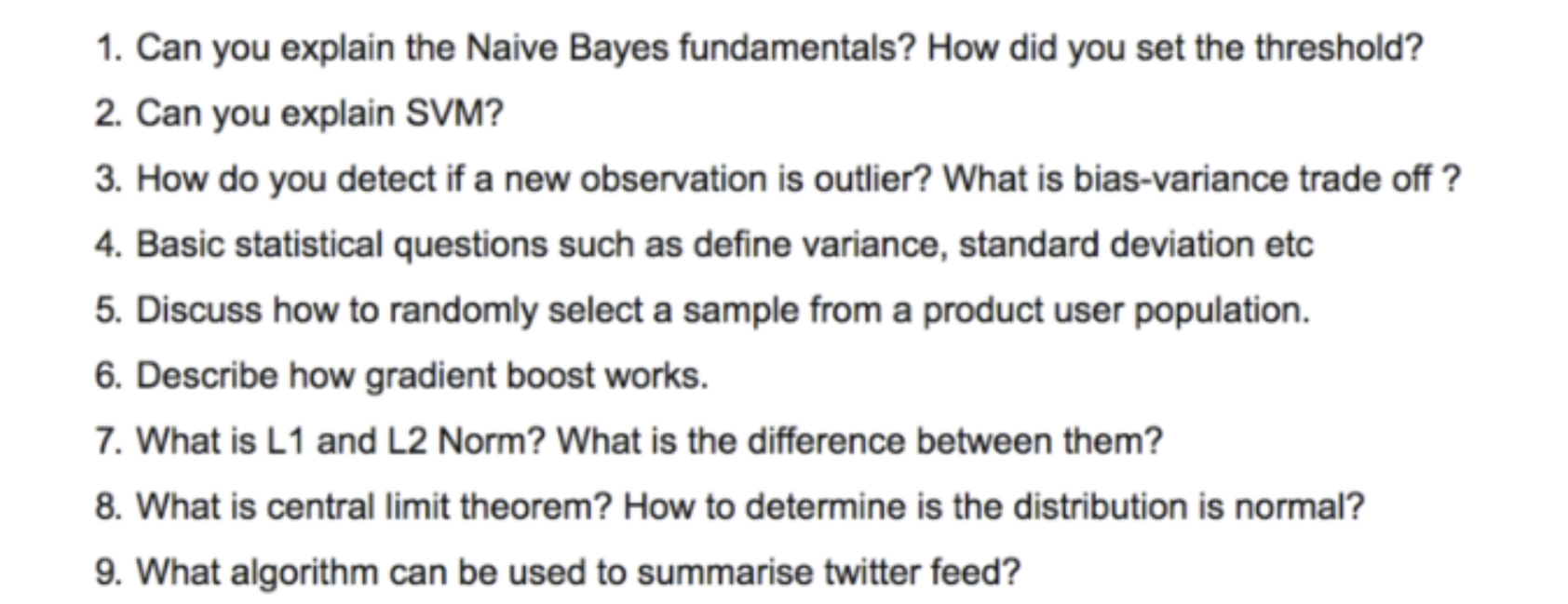Interview questions for Microsoft data science interview

Note: I cannot guarantee 100% that these were asked by Microsoft.
However, I thought that even in the case that they weren’t, this would still be a good exercise! Also, I have every right to believe that my friend provided me with valid questions.

Q: Can you explain the Naive Bayes Fundamentals? How did you set the threshold?

A: Naive Bayes is a classification model based on the Bayesian Theorem. Its biggest assumption (and why it’s called ‘Naive’) is that it assumes that features are conditionally independent given the class, which typically isn’t the case. (Thank you AlexMurphy for the clarification!)

To set the threshold, you can use cross-validation to determine the accuracy of a model based on a number of thresholds. However, depending on the scenario, you might want to take into consideration false-negatives and false-positives. For example, if you were trying to classify cancer tumors, ideally you’d want to ensure that there are no false-negative results (model says there isn’t a cancer tumor when there is).

Q: Can you explain SVM?

A: SVM stands for Support Vector Machine and is a supervised machine learning model commonly used as a non-probabilistic binary classifier , but can also be used for regression too. Focusing on the simplest use case, classifying between one of two categories, SVMs find a hyperplane or a boundary between the two classes of data that maximizes the margin between the two classes (see below). This hyperplane is then used to decide whether new data points fall under one category or the other.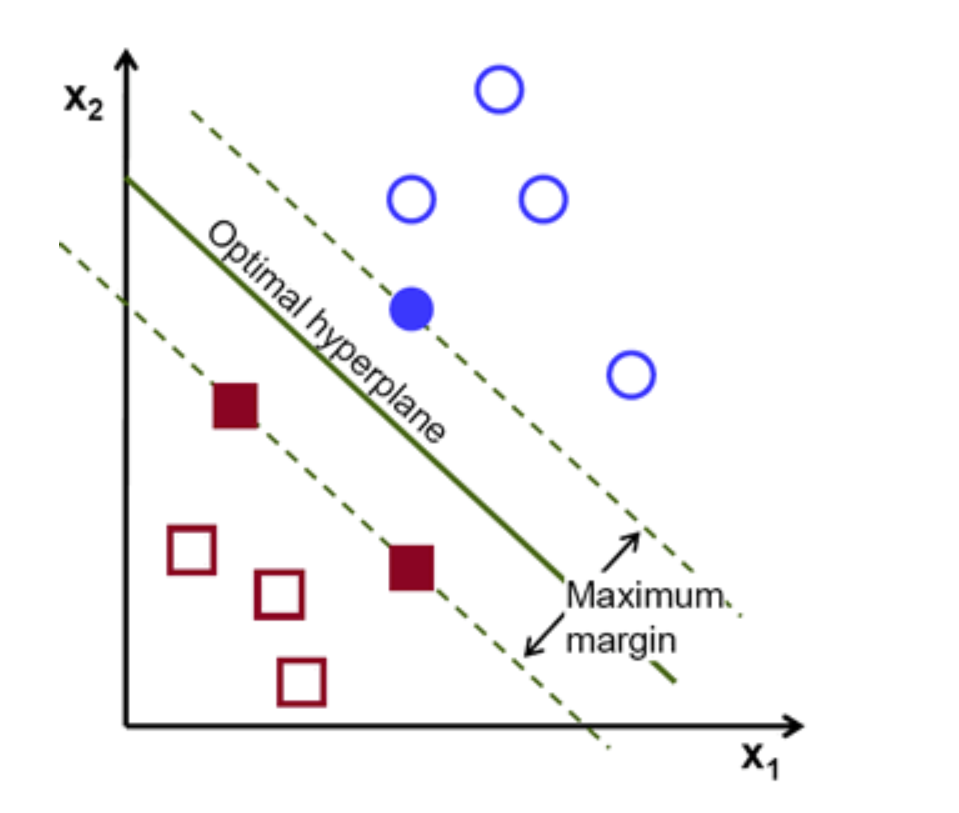Example of hyperplane separating two classes of data

However, the hyperplane is usually never as obvious and linear as the image above. Sometimes, the hyperplane can be hard to determine and rather non-linear. This is when more complicated topics like kernel functions, regularization, gamma, and margin come into play.

Q: How do you detect if an observation is an outlier?

A: There are two common methods used to determine if an observation is an outlier:

Z-score/standard deviations:
if we know that 99.7% of data in a data set lie within three standard deviations, then we can calculate the size of one standard deviation, multiply it by 3, and identify the data points that are outside of this range. Likewise, we can calculate the z-score of a given point, and if it’s equal to +/- 3, then it’s an outlier.
Note: that there are a few contingencies that need to be considered when using this method; the data must be normally distributed, this is not applicable for small data sets, and the presence of too many outliers can throw off z-score.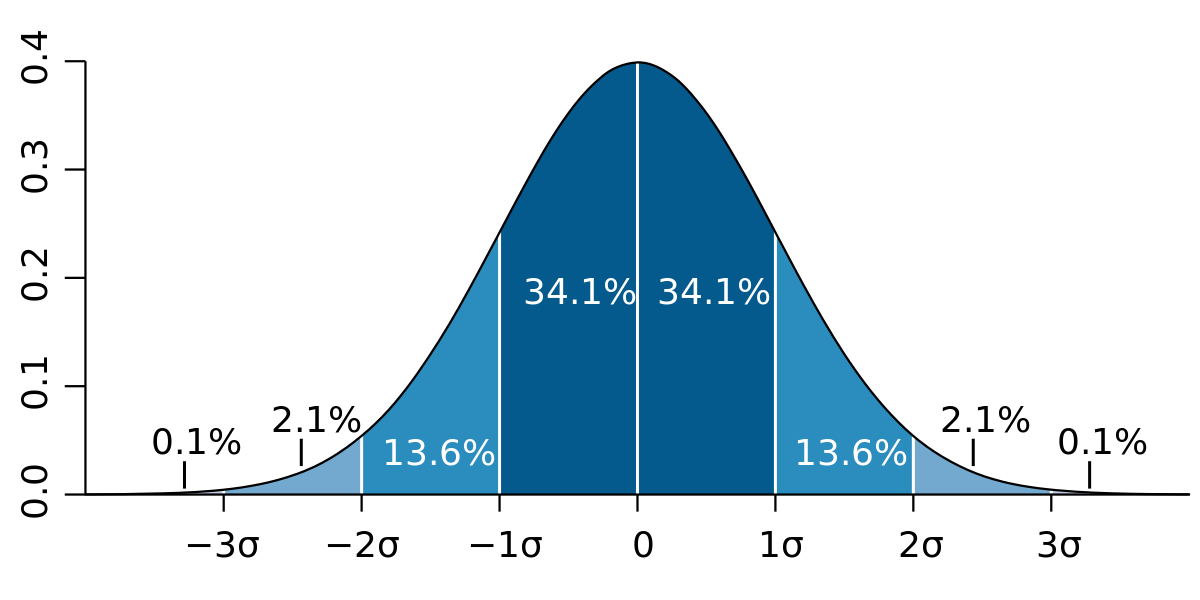Interquartile Range (IQR):
IQR, the concept used to build boxplots, can also be used to identify outliers. The IQR is equal to the difference between the 3rd quartile and the 1st quartile. You can then identify if a point is an outlier if it is less than Q1–1.5*IRQ or greater than Q3 + 1.5*IQR. This comes to approximately 2.698 standard deviations.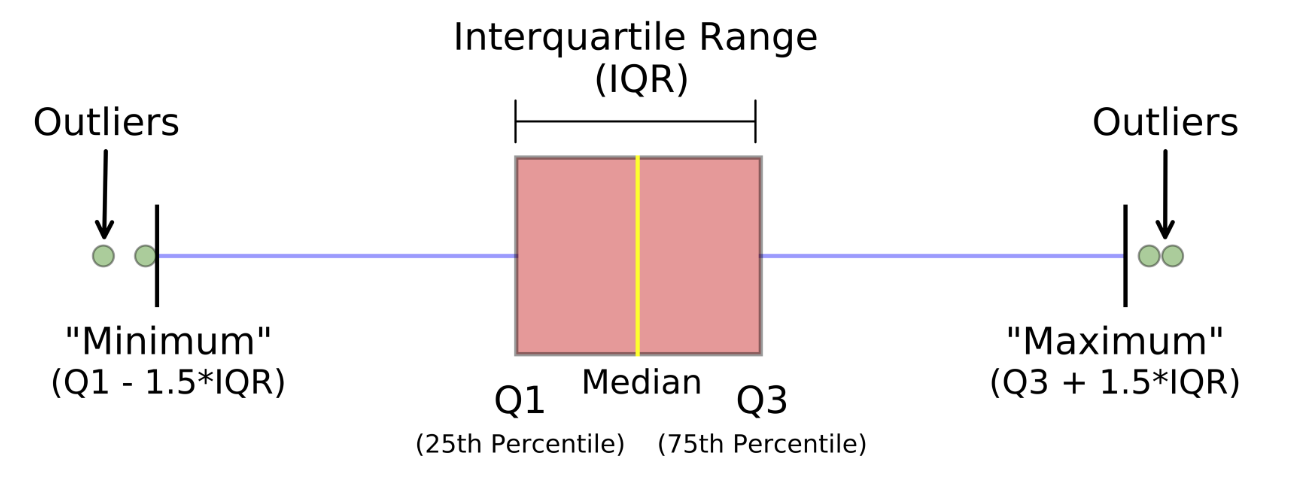Photo from Michael Galarnyk

Other methods include DBScan clustering, Isolation Forests, and Robust Random Cut Forests.

Q: What is the bias-variance tradeoff?

A: Bias represents the accuracy of a model. A model with a high bias tends to be oversimplified and results in underfitting. Variance represents the model’s sensitivity to the data and the noise. A model with high varianceresults in overfitting.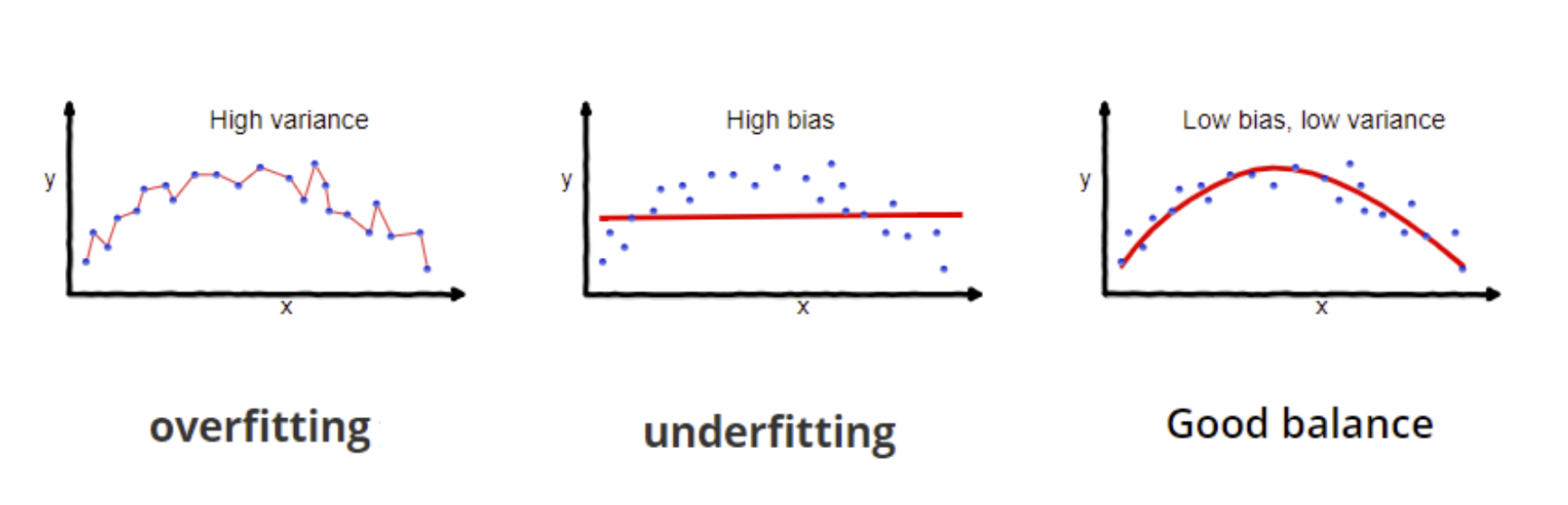Photo from Seema Singh

Therefore, the bias-variance tradeoff is a property of machine learning models in which lower variance results in higher bias and vice versa. Generally, an optimal balance of the two can be found in which error is minimized.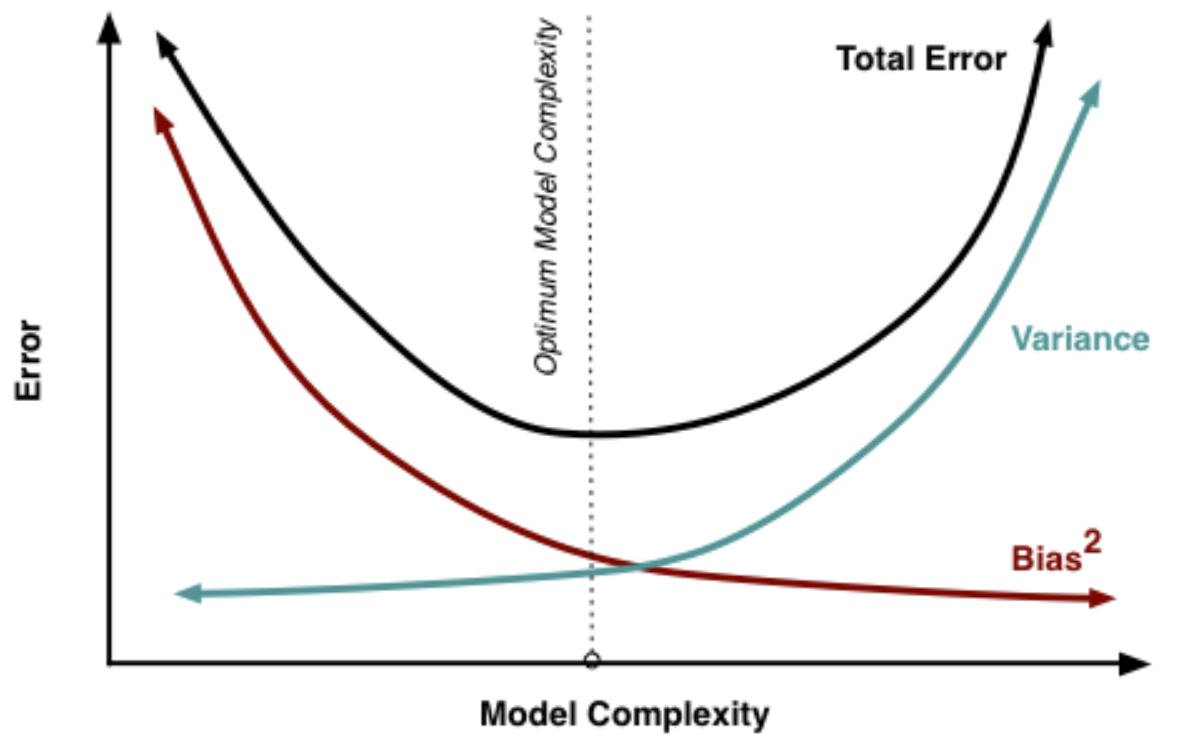Q: Basic statistical questions such as variance, standard deviation, etc…

A: Variance and standard deviation both measure how spread out a data set is relative to its mean. The difference is the standard deviation is the square root of variance.

here.

Q: Discuss how to randomly select a sample from a product user population.

A: A technique called simple random sampling can be used. Simple random sampling is an unbiased technique that randomly takes a subset of individuals, each with an equal probability of being chosen, from a larger dataset. It is typically done without replacement.

With pandas, you can use .sample() to conduct simple random sampling.

Q: Describe how gradient boost works.

A: Gradient boost is an ensemble method, similar to AdaBoost, which essentially iteratively builds and improves on previously built trees by using gradients in the loss function. The predictions of the final model are the weighted sum of the predicted of all previous models. How it goes about improving itself model after model is a little complicated, so I’ve included some links below.

Q: What is L1 and L2 norm? What is the difference between them?

A: L1 and L2 norm are two different regularization techniques. Regularization is the process of adding additional information to prevent overfitting.

A regression model that implements L1 norm is called Lasso Regressionand a model that implements L2 norm is called Ridge regression. The difference between the two is the Ridge regression takes the square of the weights as a penalty term for the loss function, whereas Lasso regression takes the absolute value of the weights.

here.
here.

Q: What is the central limit theorem (CLT)? How to determine if the distribution is normal?

A: Statistics How To provides the best definition of CLT, which is:

“The central limit theorem states that the sampling distribution of the sample mean approaches a normal distribution as the sample size gets larger no matter what the shape of the population distribution.”Central Limit Theorem explained visually

There are three general ways to determine if a distribution is normal. The first way is visually checking with a histogram. A more accurate way of checking this is by calculating the skewness of the distribution. The third way is to conduct formal tests to check for normality — some common tests include the Kolmogorov-Smirnov test (K-S) and Shapiro-Wilk (S-W) test. Essentially, these tests compare a set of data against a normal distribution with the same mean and standard deviation of your sample.

Q: What algorithm can be used to summarize twitter feed?

A: For this question, I wasn’t sure of the answer so I reached out to my friend Richie, a Data Scientist at Bell Canada!

There are a few ways of summarizing texts, but first, understanding the question is important. ‘Summarization’ could be referring to sentiment or contents, and level and sophistication of summarization could differ. I would personally clarify with the interviewer what they’re exactly looking for, but that doesn’t mean you can’t make assumptions (which is something they want to see anyways.

Assuming that the interviewer is looking for a few examples of the most interesting representative tweets, for example, you can employ TF-IDF (
term-frequency-inverse document frequency).
For example, everyone is talking about the current state between Iran and USA, so you could imagine words like “war”, “missile”, “Trump”, etc. popping up frequently. TF-IDF is meant to give more weight (aka importance) to those more frequent words and diminishes impact from words like “the”, “a”, “is” from tweets.

If you like my work and want to support me…
1. The BEST way to support me is by following me on Medium here.
2. Be one of the FIRST to follow me on Twitter here. I’ll be posting lots of updates and interesting stuff here!
3. Also, be one of the FIRST to subscribe to my new YouTube channelhere!
6. Check out my website, terenceshin.com.

“Microsoft Data Science Interview Questions and Answers!”
– Terence Shin

### Related Stories

Jan 06, 2023

#### Top 2 Online Data Science Courses to Improve your Career in 2023

The discipline of Data Science is expanding quickly and has enormous promise. It is used in various sectors, including manufacturing, retail, healt...By Nikos_DataSource
Nov 25, 2021

#### 5 Tips To Ace Your Job Interview For A Data Scientist Opening

5 Tips To Ace Your Job Interview For A Data Scientist Opening.PNG 795.94 KBImage SourceAspiring data scientists have a bright future ahead of them....By Daniel Morales
Nov 12, 2021

#### When to Avoid Deep Learning